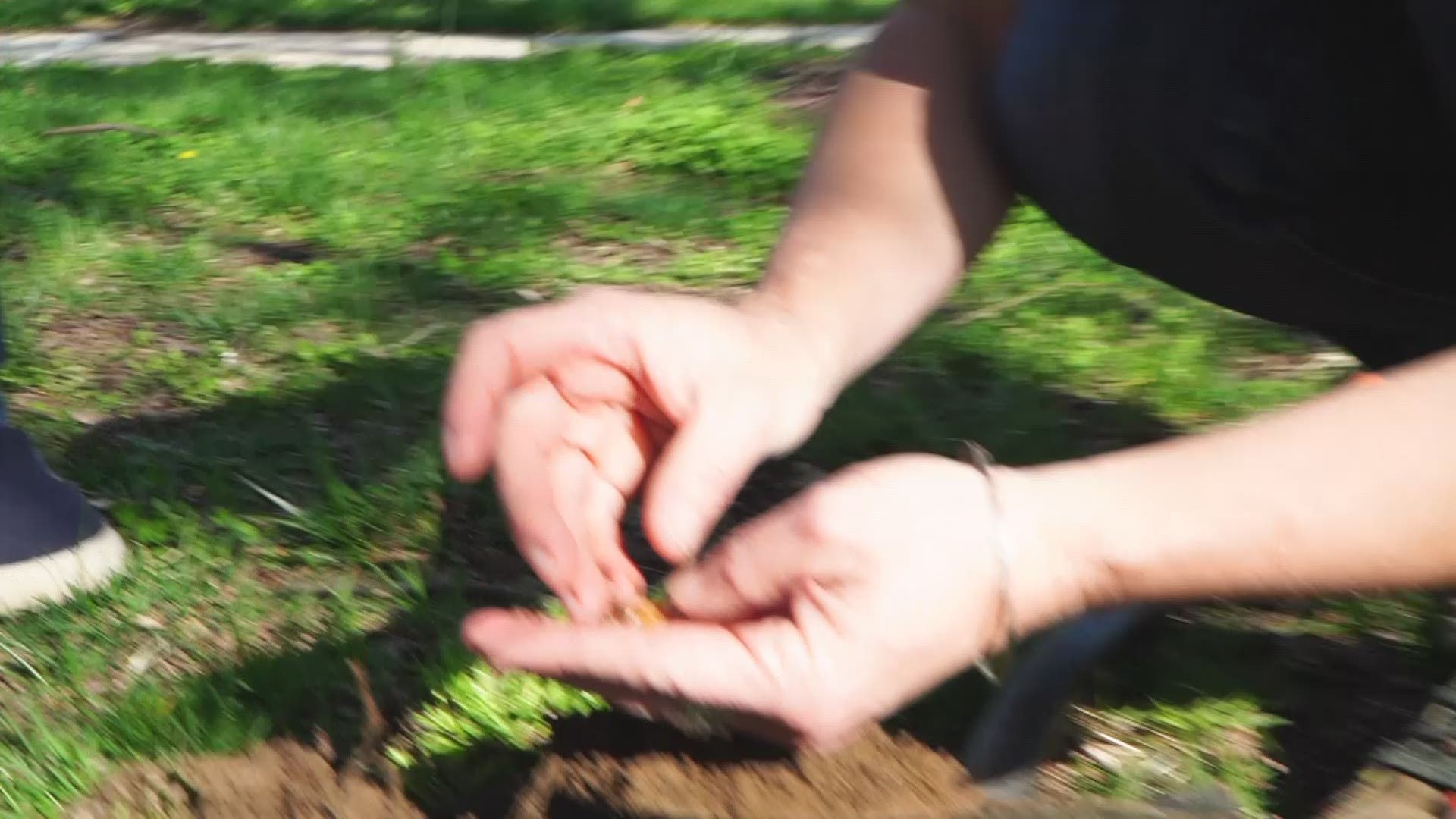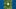Breaking News
More () »

# The magic number is 64 for the cicadas | What soil temperature means for the bugs

The onslaught of Brood X depends on soil temperature.WASHINGTON — Billions of bugs are on the way to the DMV. Brood X cicadas are expected to emerge after 17 years in May, but how can we figure out when the cicadas will actually leave the ground? Soil temperature is key.

Calculating Soil Temperature

The cicadas will start to emerge from the ground when the soil temperature reaches 64 degrees. Usually, this temperature is reached in mid-May. There is a way to use air temperature averages to estimate the current soil temperature. In fact, it is a great way to teach your kids about averages.

Take the high and low of the last three days and calculate the average temperature. First, add the high and low temperatures of day one and divide by two. That number becomes the daily average temperature of day one. Now, do the same for days two and three. The last step is to average the daily temperatures of days one, two and three.

For example, to calculate the soil temperature from Monday, April 19, 2021, we need the highs and lows on Friday, Saturday and Sunday.

Friday: 62 degrees and 43 degrees

Saturday: 62 degrees and 42 degrees

Sunday: 48 degrees and 47 degrees

So, the average daily temperature of Friday was 52.5 (62 + 43 = 105. Then, 105 divided by 2 = 52.5). On Saturday, the daily average was average was 52 degrees. The daily average on Sunday was 57.5. Now, we add up the daily averages and divide by 3 for the estimated soil temperature.

So, 52.5 + 52 + 57.5 = 162 divided by 3 = 54 degrees. The soil is roughly 10 degrees away from emergence.

Yes, you can use a probe to verify if you have one. Some instant meat thermometers work just fine.Credit: tt